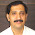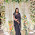### All Day Efficiency of a Transformer

All Day Efficiency of a Transformer
For a transformer, the efficiency is defined as the ratio of output power to input power. This is its efficiency. But power efficiency is not the true measure of the performance of some special types of transformers such as distribution transformers.
Distribution transformer serve residential and commercial loads. The load on such transformers vary considerably during the period of the day. For most period of the day these transformers are working at 30 to 40 % of full load only or even less than that. But the primary of such transformers is energised at its rated voltage for 24 hours, to provide continuous supply to the consumer. The core loss which depends on voltage, takes place continuously for all the loads. But copper loss depends on the load condition. For no load, copper loss is negligibly small while on full load it is at its rated value. Hence power efficiency can not give the measure of true efficiency of such transformers. in such transformers, the energy output is calculated in kilo watt hour (kWh). Then ratio of total energy output to total energy input (output + losses) is calculated. Such ratio is called energy efficiency or All Day Efficiency of a transformer. Based on this efficiency, the performance of various distribution transformers is compared. All day efficiency is defined as,

While calculating energies, all energies can be expressed in watt hour (Wh) instead of kilo watt hour (kWh).
Such distribution transformers are designed to have very low core losses. This is achieved by limiting the core flux density to lower value by using a relative higher core cross-section i.e. larger iron to copper weight ratio. The maximum efficiency in such transformers occurs at about 60-70 % of the full load. So by proper designing, high energy efficiencies can be achieved for distribution transformers.
The calculation of all day efficiency for a transformer are illustrated in the Ex. 1.

Example 1 : A 400 KVA, distribution transformer has full load iron loss of 2.5 kW and copper loss of 3.5 kW. During a day, its load cycle for 24 hours is,
6 hours 300 kW at 0.8 p.f.
10 hours 200 kW at 0.7 p.f.
4 hours 100 kW at 0.9 p.f.
Determining its all day efficeincy.
Solution : Given values are,
Pi = 2.5 kW,          (Pcu ) F.L. = 3.5 kW,          400 KVA
Iron losses is constant for 24 hours. So energy spent due to iron losses for 24 hours is,
Pi = 2.5 x 24 hours = 60 kWh
Total energy output in a day from given load cycle is,
Energy output = 300 x 6 hours + 200 x 10 hours + 100 x 4 hours
= 4200 kWh
To calculate energy spent due to copper loss,
i) Load 1 of 300 kW at cos Φ = 0.8
...                KVA supplied = kW/cos Φ = 300/0.8 = 375 KVA
...                n = load KVA/ KVA rating = 375/400 = 0.9375
Copper losses are proportional to square of KVA ratio i.e. n2.
...               Load 1 Pcu = n2 x (Pcu) F.L. = (0.9375)2 x 3.5
= 3.076 kW
...               Energy spent = 3.076 x 6 hours = 18.457 kWh
ii) Load 2 of 200 kW at cos Φ = 0.7
...                  KVA supplied = kW/cos Φ = 200/0.7 = 285.7142 KVA
...                  n = Load KVA/KVA rating = 285.7142/400 = 07142
...                 Load 2 Pcu = nx (Pcu) F.L. = (0.7142)2 x 3.5
= 1.7857 kW
...                 Energy spent = 1.7857 x 10 = 17.857 kWH
iii) Load 3 of 100 kW at cos Φ = 0.9
...                 KVA supplied = kW/cos Φ = 100/0.9 = 111.111 KVA
...                  n = 111.111/400 = 0.2778
...                  Load 3 Pcu = nx (Pcu) F.L. = (0.2778) x 3.5 = 0.2701 kW
...                   Energy spent = 0.2701 x 4 = 1.0804 kWh
iv) No load hence negligible copper losses.
...                   Total energy spent = Energy spent due to (Iron loss + Total copper loss)
= 60 + 18.457 + 17.857 + 1.0804 = 97.3994 kWh
and                  Total output = 4200 kWh
Sponsored links :

#### 11 comments:

1.2.This is something which is very interesting and lovely posting. I like all the machinery part so i like this blog.
Distribution Transformer

3.Thanks for sharing this- nice stuff! It is really nice... Great post! Thanks you so much. It is indeed a helpful one. Custom Transformer | Distribution Transformer

4.Hi, thanks for sharing the valuable formula on deciding the efficiency of a transformer. Nice info that will help me to choose the best Industrial Stabilizer manufacturers in India

5.Nice and helpful

6.7.8.There are a wide range of transformers available in the market, some of them namely – toroidal, isolation, audio output, three phase, E&I, audio line matching, SMPS and UI transformers. All these transformers have different properties and are designed for specific purposes.
Power Transformer Manufacturing Company in India | Transformer Manufacturing Company in India

9.thanks for this material...
Really it was very helpfull

10.11.